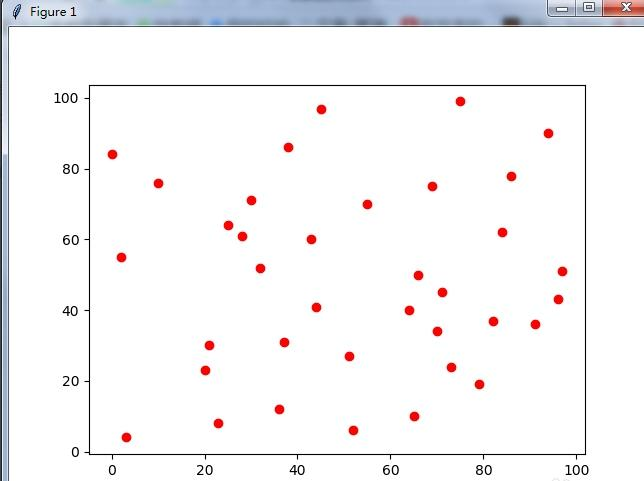# python怎么用画散点图？•  Ly   2020-05-23 16:35:342851、加载模块：

```import matplotlib.pyplot as plt
import random```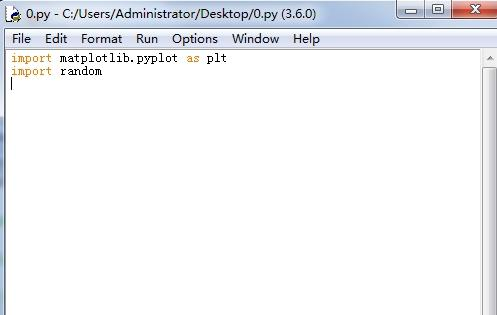2、从1到100里面，随机选取36个数字：

```a = range(0,100,1)
b = random.sample(a, 36)
print(b)```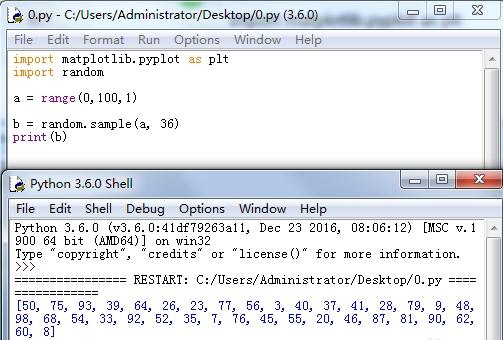3、虽然c和b的写法相同，但是运行结果却不一样，因为随机啊。

```b = random.sample(a, 36)
c = random.sample(a, 36)
print(b)
print(c)```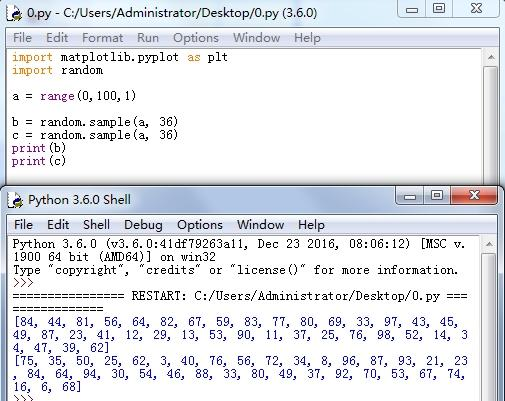4、绘制散点图：

```plt.scatter(b,c)
plt.show()```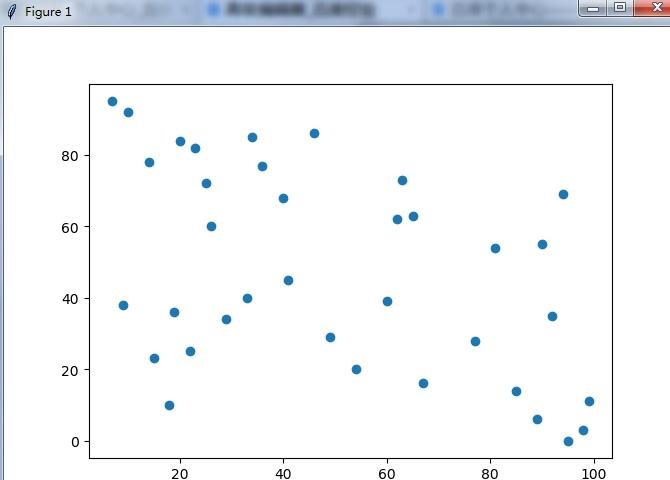5、改变点的颜色：

```plt.scatter(b,c,color = 'red')
plt.show()```